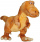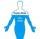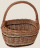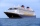# Fraction calculator

The calculator performs basic and advanced operations with fractions, expressions with fractions combined with integers, decimals, and mixed numbers. It also shows detailed step-by-step information about the fraction calculation procedure. Solve problems with two, three, or more fractions and numbers in one expression.

## Result:

### 3/4 * 1/2 = 3/8 = 0.375

Spelled result in words is three eighths.

### How do you solve fractions step by step?

1. Multiple: 3/4 * 1/2 = 3 · 1/4 · 2 = 3/8
Multiply both numerators and denominators. Result fraction keep to lowest possible denominator GCD(3, 8) = 1. In the following intermediate step, the fraction result cannot be further simplified by canceling.
In other words - three quarters multiplied by one half = three eighths.

#### Rules for expressions with fractions:

Fractions - use the slash “/” between the numerator and denominator, i.e., for five-hundredths, enter 5/100. If you are using mixed numbers, be sure to leave a single space between the whole and fraction part.
The slash separates the numerator (number above a fraction line) and denominator (number below).

Mixed numerals (mixed fractions or mixed numbers) write as non-zero integer separated by one space and fraction i.e., 1 2/3 (having the same sign). An example of a negative mixed fraction: -5 1/2.
Because slash is both signs for fraction line and division, we recommended use colon (:) as the operator of division fractions i.e., 1/2 : 3.

Decimals (decimal numbers) enter with a decimal point . and they are automatically converted to fractions - i.e. 1.45.

The colon : and slash / is the symbol of division. Can be used to divide mixed numbers 1 2/3 : 4 3/8 or can be used for write complex fractions i.e. 1/2 : 1/3.
An asterisk * or × is the symbol for multiplication.
Plus + is addition, minus sign - is subtraction and ()[] is mathematical parentheses.
The exponentiation/power symbol is ^ - for example: (7/8-4/5)^2 = (7/8-4/5)2

#### Examples:

subtracting fractions: 2/3 - 1/2
multiplying fractions: 7/8 * 3/9
dividing Fractions: 1/2 : 3/4
exponentiation of fraction: 3/5^3
fractional exponents: 16 ^ 1/2
adding fractions and mixed numbers: 8/5 + 6 2/7
dividing integer and fraction: 5 ÷ 1/2
complex fractions: 5/8 : 2 2/3
decimal to fraction: 0.625
Fraction to Decimal: 1/4
Fraction to Percent: 1/8 %
comparing fractions: 1/4 2/3
multiplying a fraction by a whole number: 6 * 3/4
square root of a fraction: sqrt(1/16)
reducing or simplifying the fraction (simplification) - dividing the numerator and denominator of a fraction by the same non-zero number - equivalent fraction: 4/22
expression with brackets: 1/3 * (1/2 - 3 3/8)
compound fraction: 3/4 of 5/7
fractions multiple: 2/3 of 3/5
divide to find the quotient: 3/5 ÷ 2/3

The calculator follows well-known rules for order of operations. The most common mnemonics for remembering this order of operations are:
PEMDAS - Parentheses, Exponents, Multiplication, Division, Addition, Subtraction.
BEDMAS - Brackets, Exponents, Division, Multiplication, Addition, Subtraction
BODMAS - Brackets, Of or Order, Division, Multiplication, Addition, Subtraction.
GEMDAS - Grouping Symbols - brackets (){}, Exponents, Multiplication, Division, Addition, Subtraction.
Be careful, always do multiplication and division before addition and subtraction. Some operators (+ and -) and (* and /) has the same priority and then must evaluate from left to right.

## Fractions in word problems:

• Dinner ticketsAt the spring festival, 7 out of the first 25 attendees had pre-purchased their dinner tickets. Based on this information, if 600 people attended the spring festival, then how many attendees could be expected to have pre-purchased their dinner tickets?
• Water in bodyWater in the human organism of all the compounds represented constitutes about 3/5 of the total weight. How many kilograms of water is in your body when you weigh 51 kg?
• Tablespoon of saltMrs. Monika uses 3/4 tablespoons of salt for every kilogram of ground pork. How many tablespoons of salt will she use for 3 1/2 kilograms of ground pork?
• Players - baseballThere are 20 players on each of two baseball teams. If 2/5 of the players on team 1 miss practice and 1/4 of the players on team two miss practice, how many more players from team 1 missed practice then team 2?
• Food weightStacie is a resident at the medical facility where you work. You are asked to chart the amount of solid food that she consumes. For the noon meal, today she ate 1/2 of a 3-ounce serving of meatloaf, 3/4 of her 3-ounce serving of mashed potatoes, and 1/3 o
• Two numbers and its productThe product of two numbers are 2/3. If on of them is 1/10, what is the other?
• The length 6The length of 12 pipes is 10 1/2 meters. (1) find the length of one pipe (2) also find the length of 7 pipes
• Percent calculationCalculate 8% if 44% is 32.
• EvaluateThe division of numbers \$a and \$b increase by-product of the numbers \$c and \$d
• Complicated sum minus productWhat must be subtracted from the sum of 3/8 and 5/16 to get difference equal to the product of 5/8 and 3/16?
• Expressions with variableThis is algebra. Let n represent an unknown number and write the following expressions: 1. 4 times the sum of 7 and the number x 2. 4 times 7 plus the number x 3. 7 less than the product of 4 and the number x 4. 7 times the quantity 4 more than the number
• Numbers divisionWith what number should be divided mixed number 2 3/4 to get 11/12?
• Crimson LynxCaptain Emily has a ship, the H. M. S Crimson Lynx. The ship is five furlongs from the dread pirate Umaima and her merciless band of thieves. If her ship hasn't already been hit, Captain Emily has probability 3/5 of hitting the pirate ship. If her ship ha